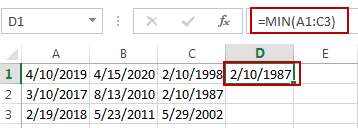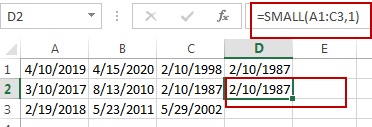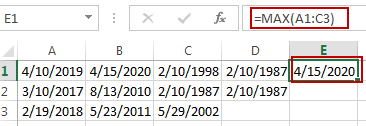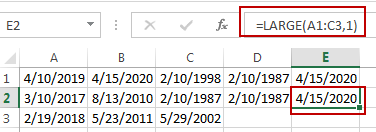# Find the Earliest and Latest Date in a Range of Dates in Excel

This post will guide you how to find the earliest date in a range of dates in Excel. How do I get the earliest and latest date in a range with a formula in Excel. How to find the highest and lowest date in a range of dates in Excel.

Assuming that you have a list of data in a range A1:C3 that contain dates, and you want to get the earliest date in these dates, how to achieve it. You can use a formula based on the MIN function or the SMALL function to get the earliest date. And if you want to get the latest date in those range of cells, you can use a formula based on the MAX function or the LARGE function.

## Find Earliest (Lowest) Date

If you want to find the earliest date in the range of cells A1:C3, just type the following formula into a blank cell, and then press Enter key.

`=MIN(A1:C3)`Or

`=SMALL(A1:C3,1)`## Find Latest Date

If you want to get the latest date in a range of cells, you can use the following formula, and type it into a blank cell, and then press Enter key.

`=MAX(A1:C3)`Or

`=LARGE(A1:C3,1)`### Related Functions

• Excel MIN function
The Excel MIN function returns the smallest numeric value from the numbers that you provided. Or returns the smallest value in the array.The MIN function is a build-in function in Microsoft Excel and it is categorized as a Statistical Function.The syntax of the MIN function is as below:= MIN(num1,[num2,…numn])….
• Excel MAX function
The Excel MAX function returns the largest numeric value from the numbers that you provided. Or returns the largest value in the array.= MAX(num1,[num2,…numn])…
• Excel LARGE function
The Excel LARGE function returns the largest numeric value from the numbers that you provided. Or returns the largest value in the array. The syntax of the LARGE function is as below:= LARGE (array,nth)…
• Excel SMALL function
The Excel SMALL function returns the smallest numeric value from the numbers that you provided. Or returns the smallest value in the array.The syntax of the SMALL function is as below:=SMALL(array,nth) …